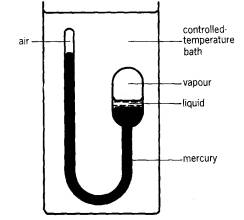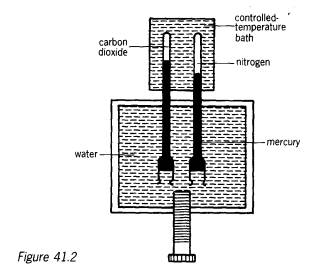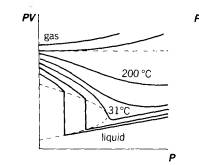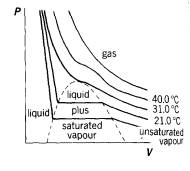Andrew experiment – alternative text: The behaviour of real gases

In our consideration of ideal gases in previous modules we have assumed that forces between molecules forces are zero and therefore that they follow the kinetic theory of gases exactly. This is not the case with actual gases, however.

A gas which follows the gas laws precisely is known as an ideal gas and one which does not is called a real gas.

In 1847 Regnault constructed PV curves up to 400 atmospheres and found that Boyle's law was not obeyed at these high pressures. Amagat went a stage further in 1892, working with nitrogen to pressures of some 3000 atmospheres (3 x 108 Pa) down a coal mine.

The idea that actual gases did not always obey the ideal gas equation was first tested by Cagniard de la Tour in 1822, using the apparatus shown in Figure 41.1.Figure 41.1

A liquid such as water or ether was trapped in a tube and the end of the tube placed in a bath whose temperature could be controlled. The temperature was then varied and the behaviour of the liquid observed. The space above the liquid is obviously filled with vapour and it was noticed that at a particular temperature no difference could be seen between the liquid and vapour states this was called the critical temperature. This phenomenon was not predicted by Boyle's law, which says nothing about the liquefaction of gases.

The classic experiment on the behaviour of gases was devised by Andrews in 1863 and used carbon dioxide as the test gas. Using the apparatus shown in Figure 41.2, he plotted a series of isothermals (PV curves) to test the validity of Boyle's law over a wide range of pressures.The gases were compressed by tightening the screw and the pressure was estimated using the nitrogen assuming that under the conditions of the experiment it still obeyed Boyle's law. The capillary tubes were very strong and Andrews obtained results up to pressures of 107 Pa.

The results are shown in the two graphs in Figure 41.3.Above about 50 oC Boyle's law was fairly closely obeyed. But as you can see, the behaviour of the gas is different above and below about 3 0 oC in fact Andrews found that the critical temperature for carbon dioxide was 30.9 oC.

Above this temperature carbon dioxide could not be liquefied by pressure alone while below this temperature an increase in pressure would finally result in liquid carbon dioxide. At the critical point the gas and liquid are in equilibrium.

These ideas form the basis for a useful definition of a vapour:

A VAPOUR IS A GAS BELOW ITS CRITICAL TEMPERATURE